• 因此在不同的场合中需要自己判断希望Precision比较高或是Recall比较高，此时我们可以引出另一个评价指标—F1-Score(F-Measure)。 F1-Score F1分数（F1 Score），是统计学中用来衡量二分类模型精确度的一种指标。它...


四个基本概念

TP、True Positive   真阳性：预测为正，实际也为正

FP、False Positive  假阳性：预测为正，实际为负

FN、False Negative 假阴性：预测与负、实际为正

TN、True Negative 真阴性：预测为负、实际也为负。

【一致判真假，预测判阴阳。】

以分类问题为例：（word公式为什么粘不过来？？头疼。）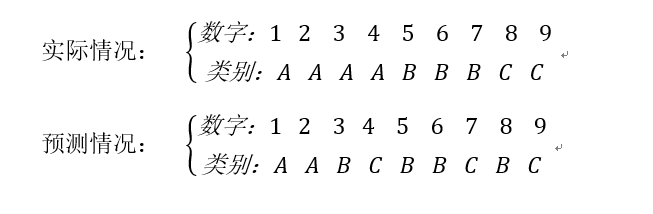首先看真阳性：真阳性的定义是“预测为正，实际也是正”，这个最好理解，就是指预测正确，是哪个类就被分到哪个类。对类A而言，TP的个位数为2，对类B而言，TP的个数为2，对类C而言，TP的个数为1。

然后看假阳性，假阳性的定义是“预测为正，实际为负”，就是预测为某个类，但是实际不是。对类A而言，FP个数为0，我们预测之后，把1和2分给了A，这两个都是正确的，并不存在把不是A类的值分给A的情况。类B的FP是2，"3"和"8"都不是B类，但却分给了B，所以为假阳性。类C的假阳性个数为2。

最后看一下假阴性，假阴性的定义是“预测为负，实际为正”，对类A而言，FN为2，"3"和"4"分别预测为B和C，但是实际是A，也就是预测为负，实际为正。对类B而言，FN为1，对类C而言，FN为1。

具体情况看如下表格：

A

B

C

总计

TP

2

2

1

5

FP

0

2

2

4

FN

2

1

1

4感谢这两位的指正

精确率和召回率计算我们预测出来的某类样本中，有多少是被正确预测的。针对预测样本而言。针对原先实际样本而言，有多少样本被正确的预测出来了。

套用网上的一个例子：

某池塘有1400条鲤鱼，300只虾，300只鳖。现在以捕鲤鱼为目的。撒一大网，逮着了700条鲤鱼，200只虾，100只鳖。那么，这些指标分别如下：

精确率 = 700 / (700 +200 + 100) = 70%

召回率 = 700 / 1400 =50%

可以吧上述的例子看成分类预测问题，对于“鲤鱼来说”，TP真阳性为700，FP假阳性为300，FN假阴性为700。

Precison=TP/(TP+FP)=700(700+300)=70%

Recall=TP/(TP+FN)=700/(700+700)=50%

将上述例子，改变一下：把池子里的所有的鲤鱼、虾和鳖都一网打尽，观察这些指标的变化。

精确率 = 1400 / (1400 +300 + 300) = 70%

召回率 = 1400 / 1400 =100%

TP为1400：有1400条鲤鱼被预测出来；FP为600：有600个生物不是鲤鱼类，却被归类到鲤鱼；FN为0，鲤鱼都被归类到鲤鱼类去了，并没有归到其他类。

Precision=TP/(TP+FP)=1400/(1400+600)=70%

Recall=TP/(TP+FN)=1400/(1400)=100%

其实就是分母不同，一个分母是预测为正的样本数，另一个是原来样本中所有的正样本数。

作为预测者，我们当然是希望，Precision和Recall都保持一个较高的水准，但事实上这两者在某些情况下有矛盾的。比如极端情况下，我们只搜索出了一个结果，且是正确的，那么Precision就是100%，但是Recall就很低；而如果我们把所有结果都返回，那么比如Recall是100%，但是Precision就会很低。因此在不同的场合中需要自己判断希望Precision比较高或是Recall比较高，此时我们可以引出另一个评价指标—F1-Score(F-Measure)。

F1-Score

F1分数（F1 Score），是统计学中用来衡量二分类模型精确度的一种指标。它同时兼顾了分类模型的精确率和召回率。F1分数可以看作是模型精确率和召回率的一种加权平均，它的最大值是1，最小值是0。（出自百度百科）

数学定义：F1分数（F1-Score），又称为平衡F分数（BalancedScore），它被定义为精确率和召回率的调和平均数。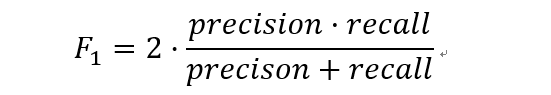更一般的，我们定义Fβ分数为：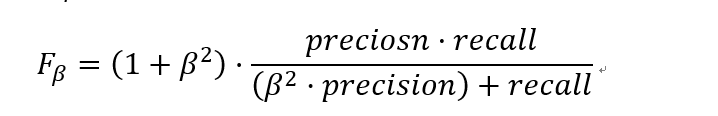除了F1分数之外，F0.5分数和F2分数，在统计学中也得到了大量应用，其中，F2分数中，召回率的权重高于精确率，而F0.5分数中，精确率的权重高于召回率。

Micro-F1和Macro-F1

最后看Micro-F1和Macro-F1。在第一个多标签分类任务中，可以对每个“类”，计算F1，显然我们需要把所有类的F1合并起来考虑。

这里有两种合并方式：

第一种计算出所有类别总的Precision和Recall，然后计算F1。

例如依照最上面的表格来计算:Precison=5/(5+4)=0.556,Recall=5/(5+4)=0.556，然后带入F1的公式求出F1，这种方式被称为Micro-F1微平均。

第二种方式是计算出每一个类的Precison和Recall后计算F1，最后将F1平均。

例如上式A类：P=2/(2+0)=1.0，R=2/(2+2)=0.5，F1=(2*1*0.5)/1+0.5=0.667。同理求出B类C类的F1，最后求平均值，这种范式叫做Macro-F1宏平均。

本篇完，如有错误，还望指正。

展开全文机器学习 数学基础
• ## #机器学习 Micro-F1和Macro-F1详解

千次阅读 多人点赞 2020-04-26 22:22:16
F1-score：是统计学中用来衡量二分类模型精确度的一种指标，用于测量不均衡数据的精度。它同时兼顾了分类模型的精确率和召回率。F1-score可以看作是模型精确率和召回率的一种加权平均，它的最大值是1，最小值是0。 ...
micro-F1和macro-F1详解摘要micro-F1：macro-F1：weighted-F1调参
摘要
F1-score：是统计学中用来衡量二分类模型精确度的一种指标，用于测量不均衡数据的精度。它同时兼顾了分类模型的精确率和召回率。F1-score可以看作是模型精确率和召回率的一种加权平均，它的最大值是1，最小值是0。
在多分类问题中，如果要计算模型的F1-score，则有两种计算方式，分别为micro-F1和macro-F1，这两种计算方式在二分类中与F1-score的计算方式一样，所以在二分类问题中，计算micro-F1=macro-F1=F1-score，micro-F1和macro-F1都是多分类F1-score的两种计算方式；
假设有如下的三分类结果：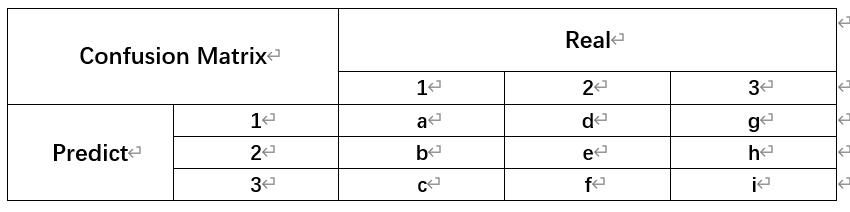根据上述结果我们可以得到一下结果（多分类中每一类都有Precision、Recall和F1-score）：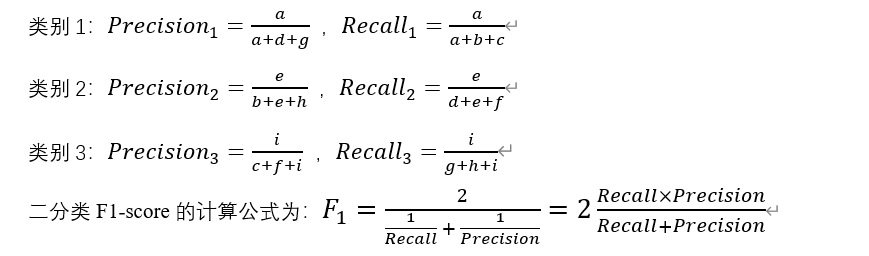两种多分类F1-score的计算方法：
micro-F1：
取值范围：(0, 1)；
适用环境：多分类不平衡，若数据极度不平衡会影响结果；
计算方式：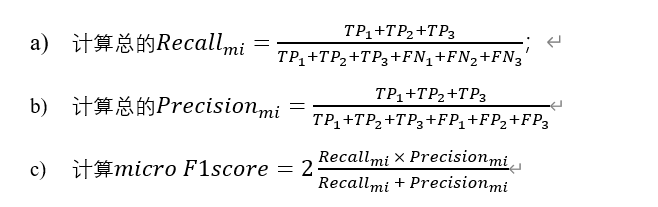TPi 是指第 i 类的 True Positive     正类判定为正类;
FPi 是指第 i 类的 False Positive   负类判定为正类;
TNi 是指第 i 类的 True Negative   正类判定为负类;
FNi 是指第 i 类的 False Negative 负类判定为负类。
假设现在有一下的三分类结果：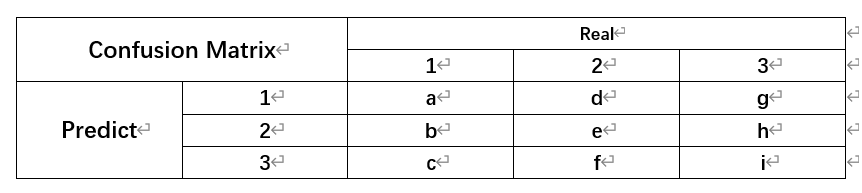由此表我们可以得出：
对第1类：FP1=d+g；TP1=a；FN1=b+c；TN1=e+f+h+i；
对第2类：FP2=b+h；TP2=e；FN2=d+f； TN2=a+c+g+i；
对第3类：FP3=c+f； TP3=i； FN3=g+h；TN3=a+b+d+e；
对micro-F1来说，mirco的计算方式使将recall和precision合起来算，所以：
对于micro-Recall：召回率为(TP1+TP2+TP3)/(TP1+TP2+TP3+FN1+FN2+FN3 )，即三个类别的TP和FN相加为分母，TP为分子。由上式分析可知，TP1+TP2+TP3 =a+e+i；FN1+FN2+FN3 =b+c+d+f+g+h（即除了TP之外的所有格），所以得到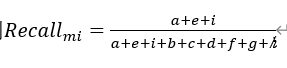对于micro-Precision：精确度为(TP1+TP2+TP3)/(TP1+TP2+TP3+FP1+FP2+FP3 )，同理可得，TP1+TP2+TP3 =a+e+i；FP1+FP2+FP3 =d+g+b+h+c+f（即除了TP之外的所有格），得到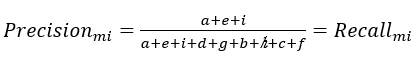然后，根据micro F1-score的计算方式可得：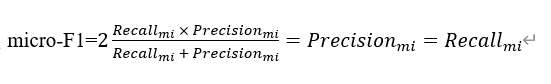而且，对于模型准确性Accuracy，定义为正确分类的样本在所有样本中的比例。所以准确性的公式
Acc=(a+e+i)/(a+b+c+d+e+f+g+h+i)
即：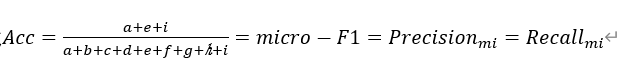据此我们可以得出结论：若micro-F1=0.5，则模型准确率Acc=0.5，Precision和Recall均为0.5，但是我们不能得出模型基本是靠猜的结论，因为若是三分类结果如下：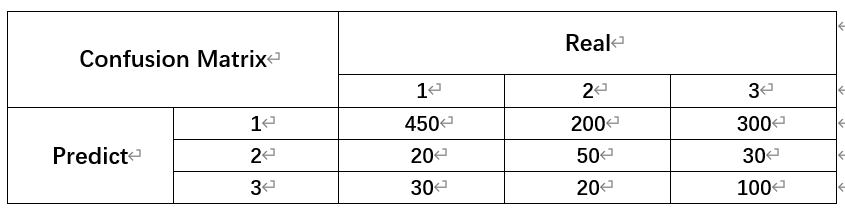Acc=(a+e+i)/(a+b+c+d+e+f+g+h+i)=micro-F1=Precision_mi=Recall_mi=0.5
macro-F1：
取值范围：(0, 1)；
适用环境：多分类问题，不受数据不平衡影响，容易受到识别性高（高recall、高precision）的类别影响；
计算方法：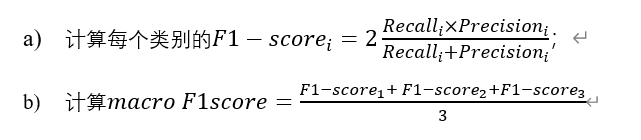macro-F1有两种计算方式，
1、先求macro-Recall和macro-Pecision，之后由这两个和求macro-F1；
2、对三个类别的F1-score直接求平均。
在sklearn的包中，使用的是第二种方式，两种方式的使用争议一直存在，但在“Training algorithms for linear text classifiers（ Lewis, David D., et al. “Training algorithms for linear text classifiers.” SIGIR. Vol. 96. 1996.）”中，作者指出，macro-F1是所有类中F1-score的平均值，即第二种方式才是macro-F1的计算方式。
我们对两种求平均的方法也可以分析，第一种方式对错误的分布不太敏感“详见论文（Opitz, Juri, and Sebastian Burst. “Macro F1 and Macro F1.” arXiv preprint arXiv:1911.03347 (2019)）”，这一点有点像micro-F1；而第二种方法则被上述论文作者推荐。
同样，我们对每一类的指标都进行分析：
对第1类：FP1=d+g；TP1=a；FN1=b+c；TN1=e+f+h+i；
对第2类：FP2=b+h；TP2=e；FN2=d+f； TN2=a+c+g+i；
对第3类：FP3=c+f； TP3=i； FN3=g+h；TN3=a+b+d+e；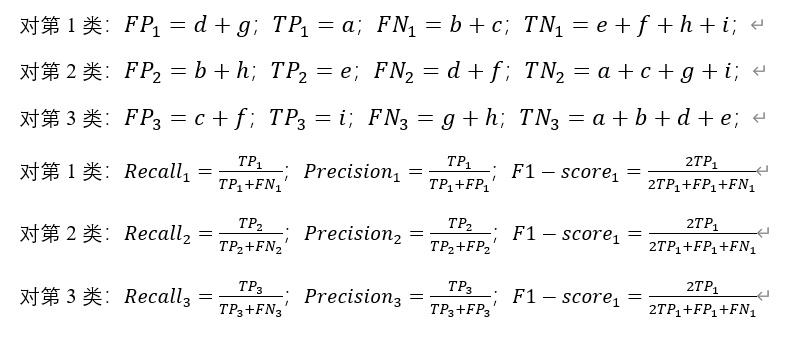将上述的值带入macro-F1中，得到：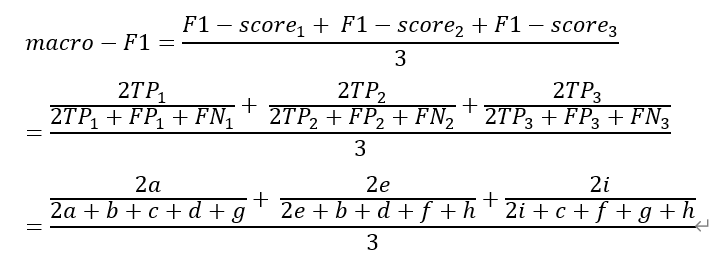上式即为macro-F1的公式（算到这里我就没有继续化简了感觉没东西）
若使该值=0.5，也得不到有用的结论。
weighted-F1
除了micro-F1和macro-F1，还有weighted-F1，是一个将F1-score乘以该类的比例之后相加的结果，也可以看做是macro-F1的变体吧。例如：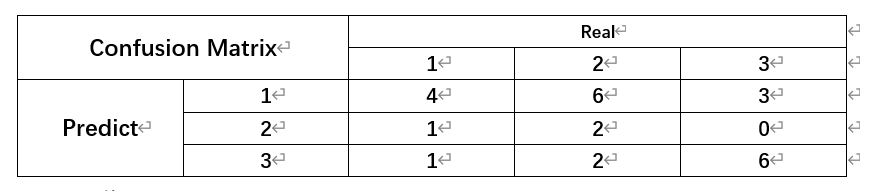对上述表格，我们可得出precision、recall和F1-score：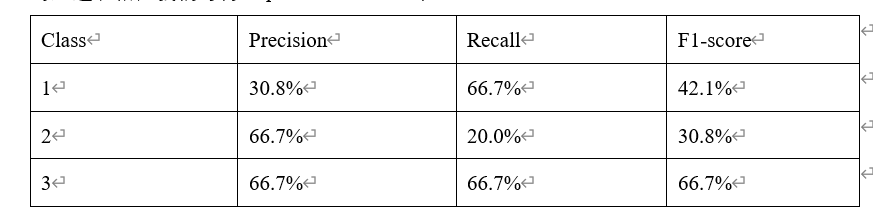所以，对weighted-F1：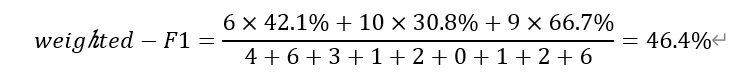同样，我们也可以算weighted-Precision和weighted-Recall：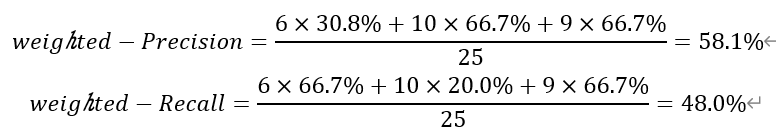其实从上面我们也可以看出来，weighted-F1并不等于由weighted-Precision和weighted-Recall计算得出的Weighted-F1(这里为了分别用大写表示)，同理，参考macro的两种计算方式。一般以第一种为结果。
weighted-F1和macro-F1的区别在于：macro-F1对每一类都赋予了相同的权重，而weighted-F1则根据每一类的比例分别赋予不同的权重。
调参
关于scoring的使用：
进行网格调参时，构建GridSearchCV，一般二分类的评分标准为roc_auc，而多分类并不提供roc曲线面积的评分方式，所以在多分类时，我们可以用
grid_search = GridSearchCV(lg, n_jobs=-1, scoring='f1_weighted', param_grid=param_dist, cv=10, verbose=5)

等评分方式来进行调参，不同的评分方式对结果都会有影响。
还有更多的评分方式可以运行这两行代码获得：
import sklearn.metrics as sm
print(sorted(sm.SCORERS.keys()))

如果错误请及时联系我，以免产生误会。
参考（需要科学上网）：


展开全文机器学习 数据分析
• API sklearn.metrics.f1_score(y_true,y_pred,labels=None,pos_label=1,average='binary',sample_weight=None,zero_division...Macro-F1: macro f1需要先计算出每一个类别的准召及其f1 score，然后通过求均值得到在...


API

sklearn.metrics.f1_score(y_true, y_pred, labels=None, pos_label=1, average='binary', sample_weight=None, zero_division='warn')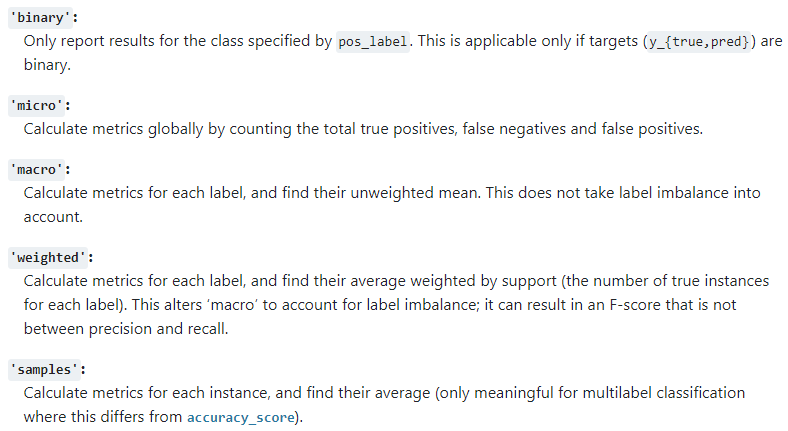Brief:

Macro-F1: macro f1需要先计算出每一个类别的准召及其f1 score，然后通过求均值得到在整个样本上的f1 score.

Micro-F1: micro f1不需要区分类别，直接使用总体样本的准召计算f1 score.

Weighted-F1: 在macro f1基础上，考虑 每一类别 的个数，进行加权平均。

Example:

from sklearn.metrics import f1_score
import numpy as np
from sklearn.metrics import confusion_matrix
from sklearn.metrics import precision_score, recall_score
y_true = np.array([0, 1, 2, 0, 1, 2, 0, 1, 0, 1, 0, 2])
y_pred = np.array([0, 2, 1, 0, 0, 1, 2, 1, 0, 1, 1, 2])

print('f1-macro', f1_score(y_true, y_pred, average='macro'),
'precision:', precision_score(y_true, y_pred, average='macro'),
'recall:', recall_score(y_true, y_pred, average='macro') )

# A    pre: 0.6   recall:0.75    f1: 0.66666
# B    pre: 0.5   recall:0.40    f1: 0.44444
# C    pre: 1/3   recall:1/3    f1:  1/3
# f1-macro
print((1/3) * (0.66666+0.44444+(1/3)) )

f1-macro 0.4814814814814814 precision: 0.4944444444444444 recall: 0.4777777777777778
0.4814777777777777

print('f1-micro', f1_score(y_true, y_pred, average='micro'),
'precision:', precision_score(y_true, y_pred, average='micro'),
'recall:', recall_score(y_true, y_pred, average='micro'))

f1-micro 0.5 precision: 0.5 recall: 0.5

print('f1-weighted', f1_score(y_true, y_pred, average='weighted'),
'precision:', precision_score(y_true, y_pred, average='weighted'),
'recall:', recall_score(y_true, y_pred, average='weighted'))

# f1-weighted
print(0.66666*(5/12) + 0.44444*(4/12) + (1/3)*(3/12))

f1-weighted 0.5092592592592592 precision: 0.5291666666666667 recall: 0.5
0.509255

confusion_matrix(y_true, y_pred)

array([[3, 1, 1],
[1, 2, 1],
[0, 2, 1]], dtype=int64)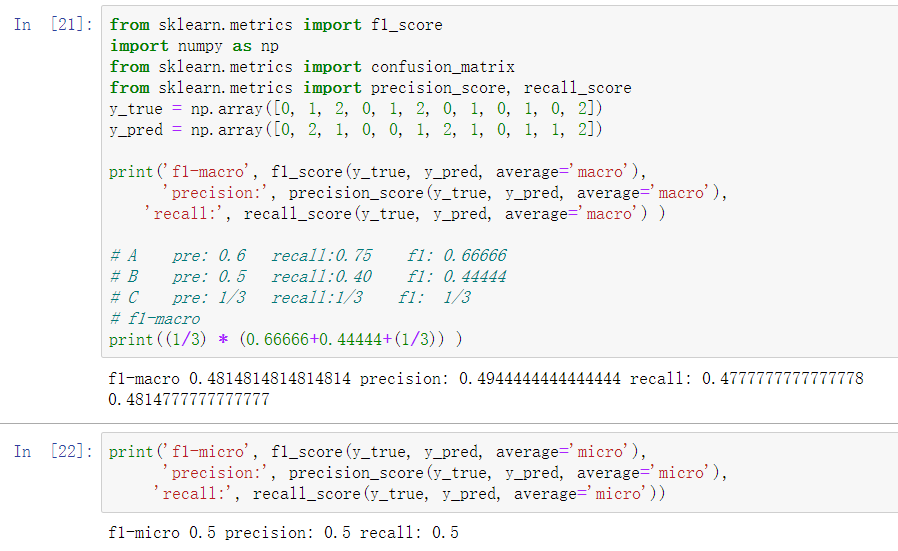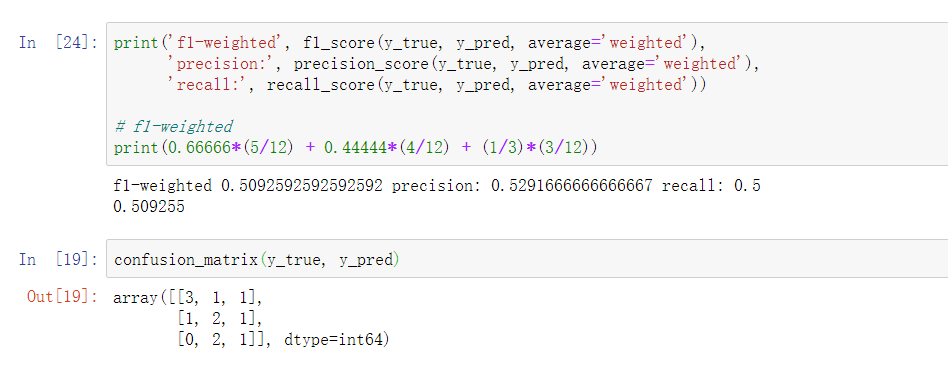展开全文• F1-Score=2∗Precsion∗RecallPrecision+Recall F1\text{-}Score=\frac{2*Precsion*Recall}{Precision+Recall} F1-Score=Precision+Recall2∗Precsion∗Recall​ 对于多分类, 因为包含多个类别, F1 得分的计算包含 ...
对于二分类, F1 得分等于 Precision 与 Recall 的调和平均数, 公式如下:
$F1\text{-}Score=\frac{2}{\frac{1}{Precision}+\frac{1}{Recall}}=\frac{2*Precsion*Recall}{Precision+Recall}$
对于多分类, 因为包含多个类别, F1 得分的计算包含 Macro-F1 和 Micro-F1 两种方式

创建示例数据
假设有 “A”, “B”, “C” 三个类别, 作了 16 次预测
import matplotlib.pyplot as plt
import numpy as np
import seaborn as sns
from sklearn.metrics import f1_score

# 3 种类别
CLASS_NAMES = ("A", "B", "C")

# 16 次预测
state = np.random.RandomState(seed=0)
# 实际标签
labels = state.randint(len(CLASS_NAMES), size=(16,))
# 预测标签
predicts = state.randint(len(CLASS_NAMES), size=(16,))

统计混淆矩阵
# 混淆矩阵
confusion = np.zeros(shape=(len(CLASS_NAMES), len(CLASS_NAMES)), dtype=np.int32)
for i in range(labels.shape):
confusion[labels[i], predicts[i]] += 1

# 打印混淆矩阵
print("混淆矩阵:", confusion, sep="\n")

结果:
混淆矩阵:
[[2 3 2]
[2 2 0]
[1 3 1]]

绘制混淆矩阵
# 绘制混淆矩阵
sns.set()

fig = plt.Figure(figsize=(6.4, 4.8))
ax: plt.Axes = fig.gca()
sns.heatmap(confusion, ax=ax, annot=True, cbar=True, fmt="d")
# X-轴与Y-轴 标签
ax.set_xlabel("Predict")
ax.set_ylabel("Actual")
# 刻度标签
ax.set_xticklabels(CLASS_NAMES)
ax.set_yticklabels(CLASS_NAMES)
# 保存可视化结果
fig.savefig("./confusion.png")

结果:样本统计(以 A 类别为例)
TP: 预测是 A 类, 实际上也是 A 类

FN: 预测不是 A 类, 实际上是 A 类

FP: 预测是 A 类, 实际上不是 A 类


A 类别

TP
FN
FP

2
3+2 (第一行除去 A)
2+1 (第一列除去 A)

B 类别

TP
FN
FP

2
2
6

C 类别

TP
FN
FP

1
4
2

Macro-F1

计算每一个类别的 Precision 和 Recall
Precision = TP / (TP + FP)
Recall = TP / (TP + FN)

A 类别:

Precision = 2 / (2+3) = 0.4
Recall = 2 / (2+5) = 0.286

B 类别:

Precision = 2 / (2+6) = 0.25
Recall = 2 / (2+2) = 0.5

C 类别:

Precision = 1 / (1+2) = 0.333
Recall = 1 / (1+4) = 0.2
# 准确率(多个类别)
class_p = confusion.diagonal() / confusion.sum(axis=0)
print("class_p:", class_p)
# 召回率(多个类别)
class_r = confusion.diagonal() / confusion.sum(axis=1)
print("class_r:", class_r)

结果
class_p: [0.4        0.25       0.33333333]
class_r: [0.28571429 0.5        0.2       ]


手动计算 Macro-F1
# ---------- 手动计算 Macro F1 ----------

# 防止分母为零
eps = 1e-6
# F1 得分(多个类别)
class_f1 = 2 * class_r * class_p / (class_r + class_p + eps)
print("各类别 F1 得分", class_f1)
# 各类别取平均值
macro_f1 = class_f1.mean()
print("Macro F1:", macro_f1)

各类别 F1 得分 [0.33333285 0.33333289 0.24999953]
Macro F1: 0.3055550891210972

sklearn 验证
print("Macro F1 in sklearn:", f1_score(labels, predicts, average="macro"))

Macro F1: 0.3055550891210972


Micro-F1

计算总体的 Precision 和 Recall, 由此得到总体的 F1-Score
总体样本的统计如下(将各个类别值相加):

TP
FN
FP

5
11
11

Precision = 5 / (5+11) = 0.3125
Recall = 5 / (5+11) = 0.3125
# ---------- 手动计算 Micro F1 ----------

# 准确率 = 召回率
p = r = confusion.diagonal().sum() / confusion.sum()
print("p or r:", p)
micro_f1 = 2 * r * p / (r + p)
print("Micro F1:", micro_f1)

结果
p or r: 0.3125
Micro F1: 0.3125

总体样本 Precision=Recall=F1-Score
sklearn 验证
print("Micro F1 in sklearn:", f1_score(labels, predicts, average="micro"))

Micro F1 in sklearn: 0.3125



展开全文机器学习
• Macro-averaging：指所有类别的每一个统计指标值的算数平均值，则对应的macro F1为多个F1值的算数平均数。 Micro-averaging：将各个混淆矩阵对应元素平均，得到TP、FP、TN、FN，然后计算相应的平均值。 weighted-...机器学习 多标签分类 评价指标
• 本文以我自己的实验数据来作为样例说明何为macro F1与micro F1 目录1 前置知识2 macro-F1与micro-F12.1 使用场景2.2 计算方法3 总结4 参考 1 前置知识 关于F1 score的内容如果还不清楚的朋友，可以参考我的上一篇...机器学习
• 3、precision、recall和F1-score 4、指标的选择问题 二、多分类评价指标 1、macro-F1 2、weight-F1 3、micro-F1 4、指标的选择问题 注：这篇文章只是对常用的分类问题中的评价指标的总结。 一、二分类评价...分类
• 【Tensorflow】利用tensor计算多分类问题的Precision、Recall、F1、Micro-F1Macro-F1 具体代码分析参考文章 logits.shape = [nodes, node_class] labels.shape = [nodes, node_class] mask.shape = [1, nodes] ...tensorflow 深度学习
• 什么是micro-F1macro-F1 (顺便谈谈精准率与召回率) TP: true positive, 预测和实际相同；（预测和真实都是A） FP: false positive, 预测为正，实际为负；（预测是A，真实不是A） FN: false negative, 预测为负，...
• F1分数（F1-Score、F1-Measure），是分类问题的一个衡量指标，用于权衡Precision和Recall，被定义为精确率和召回率的调和平均数。 F1=2⋅Precision⋅RecallPrecision+Recall.{\rm F1}=2 \cdot \frac {\rm Precision...分类算法
• 5、F1-Score 6、Micro-F1和Macro-F1 在说这个几个指标之前有一个非常重要的东西叫做混淆矩阵： 我们把上述提到的这个表格称作为混淆矩阵，那么一般使用混淆矩阵干嘛呢？计算召回率、计算准确率、计算精准率。接...机器学习 深度学习 人工智能 算法 大数据
• 在利用K_means 聚类、LR、SVM 分类，评估Embedding 结果的好坏时，遇到如下代码，不理解当中 micro_f1macro_f1 的含义，开此篇学习记录。 def classification(x, y, method='XGBoost'): x_train, x_valid...
• print('宏平均F1-score:',metrics.f1_score(y_test,y_predict,labels=[1,2,3,4],average='macro'))#预测宏平均f1-score输出 print('微平均F1-score:',metrics.f1_score(y_test,y_predict,labels=[1,2,3,4],average='...
• macro_f1 = f1_score(y_true, y_pred, average="macro") micro_f1 = f1_score(y_true, y_pred, average="micro") acc = accuracy_score(y_true, y_pred) print(macro_f1) print(micro_f1) print(acc)shellpython 机器学习
• 数学定义：F1分数（F1-Score），又称为平衡F分数（BalancedScore），它被定义为精确率和召回率的调和平均数。 更一般的，我们定义Fβ分数为： 除了F1分数之外，F0.5分数和F2分数，在统计学中也得到了大量应用，其中...
• 当 时，成为F1-Score，这时召回率和精确率都很重要，权重相同。 当有些情况下我们认为精确率更为重要，那就调整 β 的值小于 1 ，如果我们认为召回率更加重要，那就调整 β的值大于1，比如F2-Score。 G分数是另一...机器学习...